# Number theory for Cryptography and Privacy Preserving Machine Learning

This is a first post in which I intend to explain the basic ingredients needed to understand the cryptography for privacy preserving machine learning topics. Here I will cover number theory, for python code check this notebook.

Privacy preserving machine learning (PPML) is a relatively new field of study that aims to protect data privacy as well as intellectual property in the field of machine learning. It tries to solve problems like avoiding your model to retain particular information, train a model on data you cannot see or even expose a model to be used for inference whilst keeping both the original data and the prediction private.

PPML has gained interest from different academic fields like information theory and cryptography. In this post I will focus on the most basic ingredients for the latter, basic number theory. My intention is to publish a series of posts so that we can eventually understand key concepts for cryptography in machine learning and secure multiparty computation.

# Divisibility and greatest common divisor

In this section I write about division of integer numbers. This will be used through all this post in one way or another. Given two natural numbers a and b (the latter nonzero) we say that b divides a if there is another integer c such that a=b*c. If, conversely we can’t find such c then if b<a we can find a relation a=b*q+r where q is called quotient and r remainder. This, in other words is what we learn at primary school, just a bit more formalised.

If there’s a number d that divides both, a and b, we also say that d is a common divisor of a and b. For instance, 2 is a common divisor of 60 and 80 since you can write 60=30*2 and 80=40*2. One way to calculate the greatest common divisor (gcd) of two integers is through the extended euclidean algorithm: given two positive integers a and b, the following equation holds

The python code to solve this equation can be found here. For instance, the solution for the pair (a, b)=(30,27) is g, u, v = (3, 1, -1) where g is the gcd. And a last definition, a and b are said to be coprime iff gcd(a, b)=1, that is the largest number that divides both is 1.

# Modular arithmetic

Modular arithmetic is a system of arithmetic for integers, where numbers “wrap around” when reaching a certain value. First we need to fix a value m and then compute the modulo over an integer a, the result is the remainder of the division. For instance, if m=7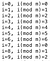Applying mod m to several i values

you can see that the value of the operation remains the same for i<m, when it reaches m it “wraps around” and begins with 0 again.

Now you may wonder… why does this have to do with cryptography? Well, there are two reasons, the first and most important is that modular arithmetic allows the construction of simple algebras like groups or fields, these are the building blocks of cryptography. The second reason is that this defines a finite set of elements (not infinite like natural numbers and real numbers) and therefore is more tractable on a computer.

The modulo operation defines an algebraic group over the sum, so if we take the previous example of m=7, the elements of the group are (0, 1, 2, 3, 4, 5, 6) and the operation is the sum modulo m. See the “multiplication” table for operation sum modulo: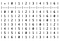sum modulo table for group defined by m=7

A group has the following properties

• Element closure: for any two elements a and b of the group, their operation returns c which is also in the group. E.g. (5+3)(mod 7)=1
• Associativity: for any three elements a, b, c it holds (a+b)+c=a+(b+c). This obviously happens in the sum operation.
• Existence of identity: There exist an element e in the set such that for any a in the set a+e=a. In this example the neutral element is 0. E.g. (5+0)(mod 7)=5.
• Inverse element: For any element in the group a there must be another element b such that a+b=e. E.g. the inverse of 5 is 2 in our example because (5+2)(mod 7)=0

If the group is commutative (i.e. a+b=b+a), then the group is also called commutative group or abelian.

## Modulo operations on product

The sum modulo operation works well to construct a group, just choose an m (any m of the natural numbers will work) and you will be in the realm of the numbers (0, 1, 2, …, m-1) and the modulo operation with addition. But what if instead of the sum we choose the product to define a group?. In this case the neutral element is 1 and we’ll find out that sometimes we cannot form a group for arbitrary m, for instance take m=10, are you able to find the multiplicative inverse of 2, i.e. find x such that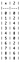multiplication table for 2 in mod 10.

From the multiplication table you can see that there’s no number that multiplied by 2 gives the neutral multiplication number (1) in the field mod 10. We can say that these elements do not form a group because we are missing inverse elements on some (if you check it, those without inverse are 2, 4, 5, 6, 8). But good news we can pick those elements that have inverse and form a group!. Let me write a multiplication table for such group in m=10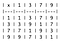Multiplication table for the group with elements (1, 3, 7, 9) and operation product modulo 10.

Here you can check by eye that all elements have another element to which if multiplied give the multiplicative identity.

Now, is there a way to know if an element has inverse modulo m? Yes!. Let a and m be integers such that a<m, then a*b (mod m)=1 for some integer b if and only if gcd(a, m)=1. Actually we can use the extended euclidean algorithm to calculate the inverse:

If a has inverse modulo m, then from the stated above: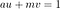Extended Euclidean equation for gcd(a, m)=1

by applying mod m to both sides of the equation we will have that

and therefore u is the inverse of a mod m. There is a special case when m is a prime number, we will denote it from now on with p. In this case, gcd(a, p)=1 since p is only divisible by himself and 1, therefore all the elements (1, 2, 3, …, p-1) will have inverse and will form a group with the product modulo p. An easy way to calculate the multiplicative inverse in a prime modulo group is to use the Fermat’s little theorem: Let p be a prime number and let a be any integer then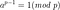Fermat’s little theorem for a not divisible by p

if a is not divisible by p, otherwise the above equation equals zero. Then to calculate the inverse of a, we just need to multiply by a^(-1) both sides of the equation.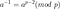Multiplicative inverse of a for a mod p field

so we can now calculate the inverse modulo p of a. This may seem heavy computationally but we use the fast powering algorithm an implementation of which can be found here.

# Rings and Fields

So far we’ve seen how to to define mathematical groups with sum and multiplication modulo an integer m. We can construct other algebraic structures using both operations. A ring is an algebraic structure consisting of a set of elements S and two operations (+, ·) that fulfil the following properties

• The set with the first operation form an abelian group. i.e. (S, +) is abelian
• There’s associativity on the second operation. I.e. if a, b, c are elements of S then (a·bc=a·(b·c).
• Existence of identity on the second operation. I.e. there exist an element e such that for any a in the set a·e=a.
• The second operation is distributive with respect to the first one. This is a·(b+c)=a·b+a·c and (b+ca=b·a+c·a

The set S={0, 1, 2, 3} with the modular operations of additions (+ mod 4) and multiplications (· mod 4) is a ring. Another example of ring is the set of matrices of dimension 3x3 and real coefficients. You can check that all properties above are accomplished (takehome exercise)

A field is a ring such that the second operation also satisfies all the abelian group properties (after throwing out the identity element of the first operation). The field has multiplicative inverses, multiplicative identity and is commutative.

A typical example of field is the set S={0, 1, 2, 3, …, p} where p is a prime number and operations are addition and multiplication modulo p. See that since p is a prime all the elements of the set have a multiplicative inverse and therefore constitute an abelian group with the multiplication operation. This field is commonly denoted as Fp. The set of matrices with dimension 3x3 and real coefficients is not a field since some matrices do not have a multiplicative inverse.

# Conclusions

We understood what is modulo arithmetic and how with such operation we can define groups, rings and fields over finite sets of elements. These structures appear constantly in cryptography, for instance when working with elliptic curves or in simple protocols like Diffie-Hellman key exchange.

In the next posts we are going to work with the defined algebraic structures to understand key concepts on cryptography and multiparty computation to the final end of understanding a bit more of privacy preserving machine learning.

Thank you for reading!. If you like the article, please star my github repo and give me some claps in the article.

PhD in Physics, AI Research Engineer

## More from Sebastia Agramunt-Puig

PhD in Physics, AI Research Engineer